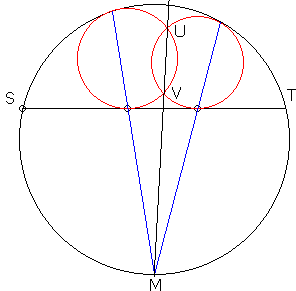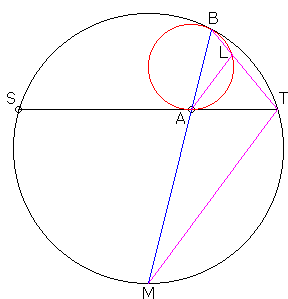### This applet requires Sun's Java VM 2 which your browser may perceive as a popup. Which it is not. If you want to see the applet work, visit Sun's website at https://www.java.com/en/download/index.jsp, download and install Java VM and enjoy the applet.

 What if applet does not run?

Explanation### The Radical Axis of Circles Inscribed in a Circular Segment

The applet suggests the following statement:

 (1) A chord ST is drawn in a circle C with center O. Two circle C' and C'' are inscribed into the circular segment thus obtained. Assume C' and C'' intersect at the points U and V and let M denote the midpoint of the arc defined by ST opposite the segment. Then U, V, and M are collinear.In order to prove (1), let's establish the following identity

 (2) MA·MB = MT2.Circles C and C' are homothetic with center B, such that A, B, and M are collinear. Triangles BTM and MAT are similar. Indeed, the two triangles share an angle at M. Also, ∠BTM equals half the measure of the arc BSM. On the other hand, ∠MAT equals half the measure of the sum of arcs BS and MT, but the latter is equal to MS. Therefore, ∠BTM = ∠MAT.

From the similarity of triangles BTM and MAT we obtain MB/MT = MT/MA, which is (2).

Now for the proof of (1). Observe that (2) holds for both C' and C'', as it does for any circle inscribed into the given circular segment. This means that M has the same power with respect to C' as it has with respect to C''. The points with that property lie on the radical axis of the two circles. The radical axis of two intersecting circles (that contains M) is the line that passes through the two points of intersection of C' and C''.

(The problem also has a simple interpretation in terms of the inversion transformation. See Problem 3 in the Inversion page.)